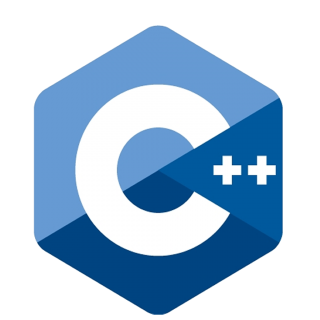11 Nov 2023
Intermediate
119 ViewsLearn via Video Course & by Doing Hands-on Labs

### C++ Programming Course

We are now well aware of what areFunctions in C++and its various aspects like function parameters, call by value and call by reference, etc. We looked at all these in detail. In this C++ tutorial, we will see how the same function can be used for different tasks i.e. C++ function overloading and its associated issues. To go a step further, take a look at our C++ Certification Course.

If two or more functions have the same name but different numbers and types of parameters, they are known as `overloaded functions`. Let us understand it by this example.
``````int area(int a) {} //for area of square
int area(int l, int b) { } //for area of rectangle
float test(float b, float h) { } //for area of triangle
``````

Here, you can see that all three functions have the same name `area()` and they perform the same operation of calculating area. The only difference is in the number and types of parameters they require to calculate the area of different shapes. Therefore, instead of defining three functions that should do the same thing, it is better to overload one. This is known as C++ Function Overloading.

Function overloading is a compile-time polymorphism technique in C++. Therefore, it becomes an important part of the OOPs concepts in C++. It promotes code reusability and understandability.

Remember: It can also happen that multiple functions have not only the same name but also the same numbers and types of parameters. This is not function overloading but `function overriding`. We will look into this after understanding Inheritance in C++ in the tutorials, Polymorphism in C++, and Function Overriding in C++ respectively.

#### Example

``````
#include <iostream>
using namespace std;

// function with 2 int type parameters
int area(int x, int y) {
return x * y;
}

// function with float type parameter
float area(float x) {
return x * x;
}

int main() {
int result1 = area(70, 2); //function call for the function with 2 int type parameters
float result2 = area(5.6); //function call for the function with float type parameter

cout << "Area of rectangle using integer arguments: " << result1 << endl;
cout << "Area of square using a float argument: " << result2 << endl;

return 0;
} ``````

The above C++ code overloads the `area()` function to calculate the area of a rectangle and square withdifferent types of parameters. Based on the type of arguments in the `function call`, the corresponding function is called.

#### Output

``````Area of rectangle using integer arguments: 140
Area of rectangle using float arguments: 47.04
``````

#### Example

``````
#include <iostream>
using namespace std;

// function with 2 float type parameters
float area(float x, float y) {
return x * y;
}

// function with a float type single parameter
float area(float x){
return x * x;
}

int main() {
float result1 = area(5.6, 8.4); //function call for the function with 2 float type parameters
float result2 = area(3.5); //function call for the function with a float type single parameter
cout << "Area of rectangle using float arguments: " << result1 << endl;
cout << "Area of square using a float argument: " << result2 << endl;

return 0;
}
``````

The above C++ code overloads the `area()` function to calculate the area of a rectangle and square with the same types of parameters but having different numbers. Based on the typeand number of arguments in the `function call`, the corresponding function is called.

#### Output

``````Area of rectangle using integer arguments: 140
Area of rectangle using float arguments: 47.04
Area of square using a float argument: 12.25
``````

## Points to Remember

• C++ function overloading is not only a feature of user-defined functions in C++
• Many standard library functions in C++ are also overloaded. E.g. the `sqrt()` function can take double, float, int, etc. as parameters. This is possible because the `sqrt()` function is overloaded in C++.
• The return type of the overloaded functions may or may not be the same.
• The parameters for each function must be in a distinct order.
• The parameters for the functions must be distinct.

• We can create functions with distinct purposes but with the same name by using `function overloading` in C++.
• It speeds up the program's execution.
• The code is clearer and simpler to comprehend.
• It reduces memory utilization and makes programs reusable.